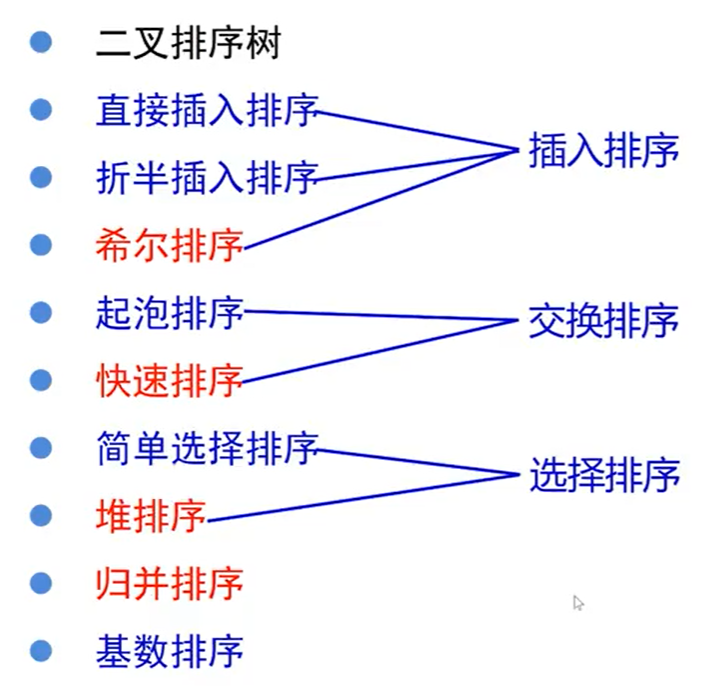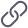• 算法的时间复杂度分析
千次阅读
2019-03-19 21:02:21

### 一、算法复杂度

算法是为求解一个问题需要遵循的、被清楚指定的简单指令的集合，简单来说就是解决特定问题求解步骤的描述。对于一个问题，一旦某种算法给定并且是正确的，那么重要的一步，就是确定该算法将需要多少时间或者空间等资源量的问题。如果一个问题的求解算法竟然需要长达一年的时间，那么这种算法就很难有什么用处了，同样，一个需要若干个GB内存的算法在当前大多数机器上也是无法使用的。

算法复杂度分为时间复杂度和空间复杂度

• 时间复杂度是指执行这个算法所需要的计算工作量
• 空间复杂度是指执行这个算法所需要的内存空间

### 二、时间复杂度

1.时间复杂度的概念

首先分析算法的时间复杂度，使用大O表示法，其核心思想是：所有代码的执行时间与代码的执行次数成正比，可以使用以下公式来表达：

                                T(n) = O( f(n) )

对该公式的解释如下：

• T(n) 表示算法中语句的执行次数
• n 表示数据规模的大小，数据规模n不同，代码的执行时间T(n)也会随之而改变
• f(n)  算法的基本操作(执行次数最多的那条语句)重复执行的次数

在进行算法分析时，代码的总执行时间T(n)是关于问题规模n的函数，进而分析T(n)随n的变化情况并确定T(n)的数量级。算法的时间复杂度，记作：T(n)= O(f(n))。它表示随问题规模n的增大，算法执行时间的增长率和f(n)的增长率相同，大O时间复杂度实际上并不具体表示代码的真正执行时间，而是表示代码执行时间随数据规模增长的变化趋势，所以称作算法的渐近时间复杂度，简称为时间复杂度。

2.分析时间复杂度的过程

2.1 计算时间复杂度遵守的守则：

（1）对于一些简单的输入输出语句或赋值语句,近似认为需要O(1)时间，（注意：常数项忽略不计）

（2）对于顺序结构,需要依次执行一系列语句所用的时间可采用大O下"求和法则"

加法原则:总复杂度等于量级最大的代码复杂度，即忽略低阶项，高阶项系数以及常数项

因此，我们只关注循环次数最多的代码

（3）对于选择结构,如if语句,它的主要时间耗费是在执行then字句或else字句所用的时间,需注意的是检验条件也需要O(1)时间

（4）对于循环结构,循环语句的运行时间主要体现在多次迭代中执行循环体以及检验循环条件的时间耗费,一般可用大O下"乘法法则"

乘法原则：嵌套代码的复杂度等于嵌套内外代码的复杂度乘积

（5）对于复杂的算法,可以将它分成几个容易估算的部分,然后利用求和法则和乘法法则技术整个算法的时间复杂度

2.2 计算时间复杂度步骤：

第一步：找出算法中的基本语句（执行次数最多的那条语句），通常是最内层循环的循环体。

第二步：计算基本语句的执行次数的数量级

第三步：将基本语句执行次数的数量级放入大Ο记号中

2.3常见时间复杂度举例

2.3.1 常数阶0(1)

int a=3;
int b=4;
int sum=a+b;

总结：只要代码的执行时间不随n的增大而增大，这样的代码时间复杂度都为O(1），一般情况下，只要代码中不存在循环语句、递归语句，即使代码成千上万，都是常数阶

2.3.2 线性阶O(n)

 int sum=0；
for(i=0;i<n;i++){
sum++;
}

2.3.3平方阶O(n^2）

最常见的平方阶算法即为两个循环嵌套的情况

int i;
for(i = 0 ; i < n ; i++){
for(j = 0 ; j < n ; j++){
System.out.println("hello");
}
}

以下这段代码的时间复杂度同样为平方阶

int i;
for(i = 0 ; i < n ; i++){
for(j = i ; j < n ; j++){
System.out.println("hello");
}
}

这段代码的内循环 j 是从i开始的，而不是从0开始，因此它的总执行时间为：n+(n-1)+(n-2)+...+1 =n^2/2+n/2

忽略低阶项，去掉最高阶系数，得出它的时间复杂度为0(n^2)

2.3.4 对数阶O(logn)

int i = 1;
while(i <= n){
i *= 2;
}

这段代码表示：当 x个2相乘大于n时，退出循环，即2^x=n    x=log2(n)  ,代码执行次数x为log2(n),

2.3.5 线性对数阶 O(nlogn)


for(int i = 0 ; i < n ; i++){
fun (i);
}

void fun(int n){
while(i<n){
i*=2;
}
}

外层循环调用的fun()函数时间复杂度为O(logn），利用乘法原则，代码的时间复杂度为O(nlogn)

2.4常见时间复杂度比较

O(1) < O(logn)  < O(n)  < O(nlogn) < O(n^2) < O(n^3) < { O(2^n) < O(n!) < O(n^n) }

注意： O(2^n)     O(n!)     O(n^n) 这三项都是非多项式时间复杂度

当N越来越大时，它们的时间复杂度会急剧增长，因此，算法时间复杂度为以上三者时，算法效率奇低

### 三、空间复杂度

空间复杂度：一个算法在运行过程中临时占用存储空间大小的量度，记做S(n)=O(f(n))，其中，n为问题的规模，f(n)为语句关于n所占存储空间的函数。

常见空间复杂度： O(1)   O(n)

对于一个算法，其时间复杂度和空间复杂度往往是相互影响的。当追求一个较好的时间复杂度时，可能会使空间复杂度的性能变差，即可能导致占用较多的存储空间；反之，当追求一个较好的空间复杂度时，可能会使时间复杂度的性能变差，即可能导致占用较长的运行时间。

更多相关内容
• 根号n段归并排序算法时间复杂度分析过程： 1.合并 根号n向下取整 段子数组使用的是自底向上两两归并的策略 2.根号n段归并排序算法时间复杂度的数学推导
• ## Dijkstra算法时间复杂度分析

万次阅读 多人点赞 2021-02-24 20:35:04
之前一直默认Dijkstra算法时间复杂度为 o(n2)o(n^{2})o(n2)，没有思考过具体的时间复杂度，今天把这个弄清楚。 Dijkstra算法的思路与关键点 思路：广度优先 + 松弛 所有点分为两个集合SSS和TTT，SSS最开始只包括...

### 文章目录

之前一直默认Dijkstra算法时间复杂度为 o ( n 2 ) o(n^{2}) ，没有思考过具体的时间复杂度，今天把这个弄清楚。

## Dijkstra算法的思路与关键点

• 思路：广度优先 + 松弛
1. 所有点分为两个集合 S S T T S S 最开始只包括源点 s s ，剩余点都位于 T T S S 集合表示已经计算出最短路径的点集合， T T 表示尚未计算出最短路径的点集合。
2. 每次从集合 T T 中选出一个与集合 S S 距离最短的点 v v ，将点 v v 加入集合 S S 。通过点 v v 对集合 T T 中的点进行松弛，即更新 T T 中点的最短距离。
3. 不断重复此步骤2，直至T集合中无法找出与集合 S S 相邻的点。
• 关键点：每次从 T T 中选出的点，距离源点的距离一定不会被松弛，因此每次选出的点都将加入集合 S S .。

### Dijkstra算法的时间复杂度

设图中的节点数为 n n ，边个数为 m m ，平均每个点的边数 k = m / n k=m/n

算法步骤2需要执行 n − 1 n-1 次，每次从集合 T T 中选出一个与集合 S S 相距最近的点，具体实现方式有4种。

• 顺序遍历集合 T T
• 使用二叉堆作为优先队列
• 使用二项堆作为优先队列
• 使用斐波那契堆作为优先队列

前提知识：二叉堆，二项堆，斐波那契堆的各种操作时间复杂度对于Dijkstra算法，给出时间复杂度的计算公式
( n − 1 ) ∗ ( T E X T R A C T − M I N + T D E L E T E + T D E C R E A S E − K E Y ∗ k ) (n-1)*(T_{EXTRACT-MIN}+T_{DELETE}+T_{DECREASE-KEY}*k)

下面对于上述四种方式，分别计算其时间复杂度。

1. 时 间 复 杂 度 = ( n − 1 ) ∗ ( T E X T R A C T − M I N + T D E L E T E + T D E C R E A S E − K E Y ∗ k ) = ( n − 1 ) ∗ ( n + 1 + k ) = n ∗ ( n + k ) = n 2 + m = n 2 \begin{aligned} 时间复杂度&=(n-1)*(T_{EXTRACT-MIN}+T_{DELETE}+T_{DECREASE-KEY}*k) \\ &=(n-1)*(n+1+k)\\ &=n*(n+k)\\ &=n^{2}+m &=n^{2} \end{aligned}
2. 时 间 复 杂 度 = ( n − 1 ) ∗ ( T E X T R A C T − M I N + T D E L E T E + T D E C R E A S E − K E Y ∗ k ) = ( n − 1 ) ∗ ( 1 + log ⁡ n + k ∗ log ⁡ ( n ) ) = n ∗ ( 1 + k ) log ⁡ n = ( n + m ) log ⁡ n \begin{aligned} 时间复杂度&=(n-1)*(T_{EXTRACT-MIN}+T_{DELETE}+T_{DECREASE-KEY}*k) \\ &=(n-1)*(1+\log{n}+k*\log(n))\\ &=n*(1+k)\log{n}\\ &=(n+m)\log{n} \end{aligned}
3. 时 间 复 杂 度 = ( n − 1 ) ∗ ( T E X T R A C T − M I N + T D E L E T E + T D E C R E A S E − K E Y ∗ k ) = ( n − 1 ) ∗ ( log ⁡ n + log ⁡ n + k ∗ log ⁡ ( n ) ) = n ∗ ( 2 + k ) log ⁡ n = ( 2 n + m ) log ⁡ n = ( n + m ) log ⁡ n \begin{aligned} 时间复杂度&=(n-1)*(T_{EXTRACT-MIN}+T_{DELETE}+T_{DECREASE-KEY}*k) \\ &=(n-1)*(\log{n}+\log{n}+k*\log(n))\\ &=n*(2+k)\log{n}\\ &=(2n+m)\log{n}\\ &=(n+m)\log{n} \end{aligned}
4. 时 间 复 杂 度 = ( n − 1 ) ∗ ( T E X T R A C T − M I N + T D E L E T E + T D E C R E A S E − K E Y ∗ k ) = ( n − 1 ) ∗ ( log ⁡ n + log ⁡ n + k ∗ 1 ) = n ∗ ( 2 log ⁡ n + k ) = 2 n log ⁡ n + m = n log ⁡ n + m \begin{aligned} 时间复杂度&=(n-1)*(T_{EXTRACT-MIN}+T_{DELETE}+T_{DECREASE-KEY}*k) \\ &=(n-1)*(\log{n}+\log{n}+k*1)\\ &=n*(2\log{n}+k)\\ &=2n\log{n}+m\\ &=n\log{n}+m\\ \end{aligned}
展开全文堆栈
• 算法时间复杂度与初始序列无关的是：选择排序、堆排序、归并排序、基数排序 算法的排序趟数与初始序列无关的是：插入排序、选择排序、基数排序 堆排序 (1)堆是一颗完全二叉树； (2)小(大)顶堆中的每一个节点都不...
排序算法中比较次数与初始元素序列排序无关的只有选择排序和基数排序，其他的都有关。

元素的移动次数与关键字的初始排列次序无关的是：基数排序
元素的比较次数与初始序列无关是：选择排序、折半插入排序
算法的时间复杂度与初始序列无关的是：选择排序、堆排序、归并排序、基数排序
算法的排序趟数与初始序列无关的是：插入排序、选择排序、基数排序

堆排序
(1)堆是一颗完全二叉树；
(2)小(大)顶堆中的每一个节点都不小于(不大于)它的父节点；
(3)堆的插入、删除元素的时间复杂度都是O(log n)；
(4)建堆的时间复杂度是O(n)；
(5)堆排序的时间复杂度是O(nlog n)；
(6)堆排序的空间复杂度是O(1)​；展开全文堆排序 数据结构 排序算法 快速排序
• 对于我们编写的算法，我们有必要去分析时间复杂度，即分析它的运行效率，而运行效率又取决于算法的运行次数。一个好的算法，它应该是运行时间短的，占用内存空间小的，学会了如何去分析算法时间复杂度并计算出...

🤡🤡🤡接着上一篇文章《算法的时间复杂度、空间复杂度》，本篇学习简化的算法时间复杂度分析！内容不多，简简单单！🤩🤩🤩

算法的时间复杂度、空间复杂度http://t.csdn.cn/2V9Yh

🐥🐥🐥🐥🐥🐥🐥🐥🐥🐥🐥🐥🐥🐥🐥🐥🐥🐥

## 一、👻前言

对于我们编写的算法，我们有必要去分析去时间复杂度，即分析它的运行效率，而运行效率又取决于算法的运行次数。一个好的算法，它应该是运行时间短的，占用内存空间小的，学会了如何去分析算法的时间复杂度并计算出大概的结果，这有助于我们编写出更优的算法，这也是作为一个好的程序员必备的技能。

但是一个算法执行所耗费的时间，从理论上是不能算出来的，必须上机运行测试才能知道。但我们不可能也没有必要对每个算法都上机测试，只需知道哪个算法花费的时间多，哪个算法花费的时间少就可以了。

## 二、😇何为简化的算法时间复杂度分析

在通常中，我们计算一个算法的时间复杂度，都在一步一步的分析每一行代码的运行次数，然后把它们加起来，最后计算出算法频度T（n），得出时间复杂度。

但是这种方法太过于繁琐，耗时长，往往我们不会愿意花太多的时间进去这样计算算法的时间的复杂度，所以有必要简化计算的步骤。

那么何为简化？(●'◡'●)

• 仅仅考虑算法中的基本操作，即算法中最深层循环内的原操作。
• 算法执行时间大致=基本操作所需时间*其运行次数

所以在算法分析中，计算T（n）时仅仅考虑基本操作的执行次数。

例如下面这个算法：

void matrixadd(int[][] A,int[][] B,int[][] C,int n)
{
for(int i=o;i<n;i++)
for(int j=0;j<n;j++)
C[i][j]=A[i][j]+B[i][j];  //只考虑该原操作
}


我们可以看到，该算法一共两个for循环语句，而每个执行n+1次，最后一行代码为原操作，那么我们的算法频度即为T(n)=n^2

对应的时间复杂度为：O(n^2)

具体计算：

你学废了吗？小玥早就废了！！！

🐷🐷🐷♥(｡￫v￩｡)♥🐷🐷🐷

展开全文大数据 数据结构 java
• 随机选择算法时间复杂度分析 首先提供算法的伪代码： 算法是递归算法 时间复杂度分析思路：计算每一次递归语句所消耗的时间，再求和。 为了分析需要，我们先定义如下的量： 定义状态j：数组长度在[(3/4)(j+1)n,(3/4...
• ## 递归算法时间复杂度分析

万次阅读 多人点赞 2018-09-17 16:16:59
递归算法时间复杂度分析 时间复杂度： 一般情况下，算法中基本操作重复的次数就是问题规模n的某个函数f（n），进而分析f（n）随n的变化情况并确定T（n）的数量级。这里用‘o’来表示数量级，给出算法时间复杂度。 T...
• 算法时间复杂度分析 在计算机程序编写前，依据统计方法对算法进行估算，经过总结，我们发现一个高级语言编写的程序程序在计算机上运行所消耗的时间取决于下列因素: 1.算法采用的策略和方案; ⒉编译产生的代码质量; 3...
• 看了左神的求递归算法时间复杂度分析受益颇多，在这里写一下收获： master公式的使用 T(N) = a*T(N/b) + O(N^d) 1) log(b,a) > d ->复杂度为O（N^log(b,a)） 2) log(b,a) = d ->复杂度为O（N^d*logN） 3) ...迭代算法复杂度分析
• 具体点来讲就是我们在实现某一种算法的时候，最终目的就是要求计算机(CPU)在最短的时间内，用最少的内存稳定的输出正确的结果。这一章节主要来理解 “快”，至于“省” 和 “稳”，我会在后续章节进行讲解。 那如何...算法复杂度 大O表示法
• 算法时间复杂度分析基础算法时间复杂度分析基础算法时间复杂度分析基础
• 算法复杂度分为时间复杂度和空间复杂度。 其作用： 时间复杂度是指执行算法所需要的计算工作量； 而空间复杂度是指执行这个算法所需要的内存空间。 （算法的复杂性体现在运行该算法时的计算机所需资源的多少上，...
• 算法时间复杂度分析 算法采用的策略和方案 编译产生的代码质量 问题的输入规格（100和1亿是不一样的） 机器执行指令的速度 抛开计算机硬件、软件有关的因素，一个程序的运行时间依赖算法的好坏和问题的输入规格...数据结构
• 时间复杂度分析的简单规则6.两种时间复杂度7.常见的时间复杂度8.空间复杂度分析9.不同情况下的复杂度分析10.算法时间复杂度与数据规模11.总结 算法时空复杂度分析 0.为什么要学习算法时空复杂度分析 算法的复杂度...数据结构
• 算法设计与复杂度分析 算法复杂性分析 算法复杂性是算法运行所需要的计算机资源的量 需要时间资源的量称为时间复杂性,需要的空间资源的 量称为空间复杂性这个量应该只依赖于算法要解的 题的规模算法的输入和算法本身...
• 最大公约数的三种算法_复杂度分析_时间计算，代码实现复杂度分析，以及计时处理最大公约数
• 下面介绍几种递归函数中的算法时间复杂度分析的方法 0.递推法 这种方法是最简单的，也是最容易理解的一种方法，对于一个递归函数，我们可以利用他的递归方程，将其递推到常量的情况下，这样子就很容易求解他的时间...数据结构
• title: ‘[算法设计与分析-概念]算法复杂度分析’ date: 2020-09-28 21:09:32 tags: - 算法复杂度分析 categories: 算法设计与分析 #什么是复杂度分析？ 数据结构和算法解决是“如何让计算机更快时间、更省空间的...
• 复杂度分析简介 常数项不计入复杂度 当数据规模小的时候，可以考虑复杂度比较差的算法 但是当规模比较大时，我们就必须追求复杂度 时间复杂度如果由两部分组成，选取大的那部分 但是要...
• 算法分析算法的时间复杂度分析函数渐进增长算法时间复杂度大O记法常见的大O阶函数调用的时间复杂度分析最坏情况算法的时间复杂度分析常见内存占用算法空间复杂度     研究算法的目的就是如何花更少的时间，如何...java 数据结构
• 文章目录前言一、什么是时间复杂度？一种简单粗暴衡量算法时间复杂度的方法（事后统计）通过预先估算来得到算法复杂度的方法（事前...因此，作为程序员，掌握基本的算法时间复杂度分析方法是很有必要的。 一、什么是数据结构
•递归
• 如果把“递归算法”叫做“套娃算法”，或许可以减少一些恐惧程度。 套娃是有限的，同样，递归也是有限的，这和我们经常在影视作品中看到的“无限嵌套循环”是有很大区别的，递归一定存在某个可以返回的节点或条件，...
• 不过，不同的语言，不同的编译器，不同的CPU来说，对程序的处理的时间是不同的，我们无法单单用运行时间来描述某个算法执行效率。另外，当需要处理的数据增长时，算法的基本操作要重复执行的次数也会增长，对于不同......